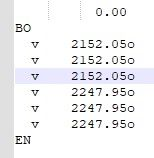# Batch Decimal Rounding

• Hello,
I need to apply in a regular basis a simple edit in a Cnc (G-Code) group of files in a given folder.
All decimal positions in them need to be rounded to cero, in a way that when the value of the first decimal after the delimeter (.) goes between 1-5 last integer before the delimiter remains the same; On the other hand, las integer increases +1 when this first decimal goes from 6-9.
There are some cases where the last position after delimiter are not numbers, but letters (shown in the inserted file)
In that cases, letters need to remain. Thnks for your help.• By itself, regular expression search-and-replaces in Notepad++ cannot do mathematical rounding. You could build a tenuous regular expression that would work in many cases, but you’d be almost guaranteed to have somewhere in your data a value that broke a limitation. I do not recommend this route.

We could write something to use with the PythonScript plugin, which would do a mathematical replacement on the active file – in that case, I’d highly recommend that you use mathematical rounding, rather than the strange rule that you just gave (which would round down for 0.01 to 0.59, and round up for 0.60 to 0.99 – which seems a strange division point; normally it would be down for 0.01-0.49, and up for 0.50-0.99 … though the exact value of 0.50 can go either way, or might use the round-to-even rule). This would use the same basic principle as the quote from the PythonScript docs which @Alan-Kilborn quoted here, something like:

``````def round_it(m):
return "{:4.00f}".format(round(float(m.group(1))))

editor.rereplace(r'\b(\d+\.\d+)', round_it)

``````

But if you have a whole directory of files, you would have to do it one file at a time, or include in the script the logic to open each file, and then run each file through that rereplace. At which point, it might be simpler to just write a command-line Python script (or whatever your favorite program language is) to do the processing without invoking Notepad++'s file opening for each file, and instead just use the language’s built-in I/O, which is going to be much more efficient.

• Clarification/correction time:

``````    return "{:4.00f}".format(round(float(m.group(1))))
``````

Whoops, sorry, that line should have been

``````    return "{:4.2f}".format(round(float(m.group(1))))
``````

… use the same basic principle as the quote…

I meant that the docs quoted gave an example of adding 1 to the matched number, and using the `editor.rereplace` method to call that add_1; similarly, here, we will use `editor.rereplace` to call `round_it` for each match.

The regex `\b(\d+\.\d+)` says “look for a boundary between spaces and numbers, and look for a number that has at least one digit before the decimal and at least one digit after, and grab that greedily, storing the number in group(1)”.

The (corrected) `round_it` function then says take group(1) from match `m` (the argument to the callback function), convert it from a string to a floating-point number, then round that floating-point number in the python definition of round (round-to-nearest, with 0.5 going away from 0), and format that rounded number as float with two digits after the decimal point. By returning that string, that is the replacement that will happen for that particular match.

Note that my regex wasn’t very restrictive; it assumed any boundary followed by something floating-point-number-like would be converted. if you wanted only after a `v` and some spaces or tabs, the regex raw-string could be something like `r'v\h+(\d+\.\d+)'` (not tested) … and if you wanted to consume the `o` after the float, then it would have to change some more. If you want more specificity in the match, you’ll have to give us better before and after data that shows some edge cases.